# Everything You Always Wanted to Know About Derivatives

## But were afraid to askPhoto by Caleb Woods on Unsplash

You’re sitting in a classroom. You look around and see your friends writing something down. It seems they are taking the exam, and they know all the answers (even Johnny who, how to say it… wasn’t the brilliant one). You realize that your exam is in front of you, and it’s Maths. You start reading it but you don’t understand a thing. That’s terrible, your heart speeds up, you’re sweating and then… you wake up.

Uff, it was only a dream. You get back to sleep, but one thing bothers you. This paper from a dream, there was something about… how is it called… derivatives? You remember you learned it by heart at school, but never truly understood it. It’s time to face this ghost from the past.

# Don’t be afraid, it’s there for a while

The idea of the derivative is not new. There is a little bit of controversy about the inventor of derivatives. The battle is between Sir Isaac Newton and Gottfried Wilhelm Leibniz.

Apparently, these two great minds discovered it independently, not being aware of their colleagues’ work. What’s interesting they came to similar conclusions having completely different ideas and approaches to the problems they were trying to solve.

Newton thought in the context of physics and motion, while Leibniz thought in terms of formula that could describe a change in the metaphysical meaning. However, people worked on similar theorems before the 17th century, when Newton & Leibniz lived. Arab and Persian mathematicians from the 11th and 12th centuries are supposed to discover basic ideas behind derivatives.

# And it’s useful!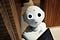Photo by Alex Knight on Unsplash

If you have ever been wondering if you’ll use any of the things you learned at school in real life, the answer is yes, derivatives are such a thing. Nowadays they are an important part of algorithms in many innovative areas, like Artificial Intelligence. In Machine Learning which is one of the AI domains, derivatives help computer programs to learn.

Generally speaking, such algorithms optimize objective functions (very often derivatives are needed for this purpose) so programs can find optimal parameters to solve different tasks (e.g. recognizing people on photos). Let’s get the key idea behind derivatives.

# And yet it moves

Leibniz was right, it’s all about the change.

The derivative of a function gives us information about how this function is changing.

Is it increasing? Decreasing? Or maybe it’s constant? How fast is it changing? In which direction? These are questions that depict the usefulness of derivatives. They also explain why Newton considered it in terms of the motion. Motion is also a change.

Ok, but to understand the change of the function, we need to understand what is the function itself. So let’s do a small step back and define a function.

You can imagine a function as a rule that transforms input and produces an output. It is required that such a rule can produce only one output for a given input. Putting it in other words, the function assigns one output value to each input value.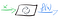Function scheme (Image by Author).

One of the simplest functions is called a linear function. Yes, it’s a straight line. Linear functions transform input number x by multiplying it by coefficient a and adding coefficient b. That’s how we compute the output of function f(x).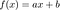Linear function formula (Image by Author).

To visualize an example let’s set parameters a and b to 1. As a result we get f(x) = 1x +1. However x multiplied by 1 is x, so finally f(x) = x + 1. If we put 0 as x we get f(0) = 1, f(1) = 2 and so on. That’s the graph of our function: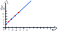Graph of f(x) = x + 1 (Image by Author).

Having in mind the key idea about derivatives and functions, let’s put it all together. We’ll see how derivative describes changes of our linear function f(x).

# Growth or decay?

To determine the change of our function f(x) = x + 1, we can take a look at its graph and calculate what’s the change on the vertical axis when the value on the horizontal axis (x) changes by one unit.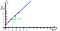Deltas (Δ) of x and f(x) (Image by Author).

As you can see in the picture, if we change x by 1 (Δx = 1), it results in grow of f(x) by 1 (Δf(x) = 1). Our line is going up, it’s increasing. And that’s exactly what a parameter tells us (a = 1).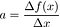“a” coefficient of a linear function (Image by Author).

If a would be negative, for example, a = -1, our line would go down. And the function would decrease by 1 unit per 1 unit change of x.

But what happens when our function is not a line, but a curve? In such cases, we need to check how our function is changing at each point that is interesting for us. The curve can increase, decrease, or be constant in different areas of the space.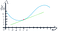Tangent (Image by Author).

To check the change of function in a given point we can think of tangent in this point of the curve (green line) and the a coefficient of this tangent. It has information about the change.

# Rules to rule them all

Graphs are a really useful form of visualizing functions, however, in more complex scenarios it’s not easy to plot a graph. Functions can have more dimensions than we’re able to see and imagine. Finally, sometimes it’s just easier to calculate the derivative that plot the function.

Fortunately, there is a set of rules we can use to calculate derivatives. Let’s introduce 3 simple yet useful ones.

# The derivative of a constant

Constant means no change at all. As a result, the derivative of something constant is equal to zero. As simple as that. This idea is compatible with our considerations regarding a coefficient of a linear function.

If a = 0, the only things that is left in the function is parameter b, so f(x) = b. And b is a constant number like 4. Let’s have a look at the graph: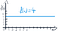A constant function f(x) = 4 (Image by Author).

Extremely stable, isn’t it?

# The derivative of a powerDerivative of a power (image by Author).

Calculating the derivative of a power is also very simple. Having function f(x)=xⁿ, we put n before x and raise x to the power of n-1. Symbol f ’(x) means the derivative of function f(x). For example:Derivative of x² (image by Author).

Actually, this function is a parabola: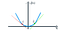Parabola slopes (image by Author).

In point A a derivative will be negative, because the tangent slope is decreasing. Our parabola goes through (0, 0), so in point A (to the left of 0) x will be negative, e.g. -5. We can calculate it:Negative derivative value for x = -5 (image by Author).

When we choose point B on the other side of the axis, x = 5, we see that tangent line is going up. Derivative at this point should be positive. Let’s check it: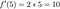Positive derivative value for x = 5 (image by Author).

# Chain rule

It sounds a little bit spooky, chains, ghosts, etc. Ok, it’s the most complex rule we learn today, but no worries, we’ll follow a simple example.

Chain rule is useful when we need to calculate a derivative of so-called composite functions. You can imagine a composite function, as a function that includes another function inside. To spark your imagination even more, composite functions are like Russian dolls.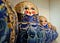Photo by Iza Gawrych on Unsplash

Having function f that contains function g is f(g(x)). We calculate the derivative of function f using this formula:Chain rule (image by Author).

Let’s decipher it. The first part of a chain rule says that we have to calculate a derivative of a function f’(g(x)), so the derivative of an “outside” function. Then we have to multiply it by a derivative of an inner function g’(x).

An example will shed some light on these chains. Let’s have a function g(x) = x² and function f(g(x)) = (x²)². It’s equal to x⁴ and using the power rule we know that (x⁴)’ is equal to 4x³. But for the sake of example let’s calculate it using the chain rule and check if we get the same result.

So the first step is to calculate the derivative of the outer function f’(g(x)), in our case it’s (g(x))². So we use a power rule and we get:Derivative of an outer function (image by Author).

The second step is to calculate the derivative of the inner function g(x), let’s use power rule again:Derivative of an inner function (image by Author).

Finally, we have to multiply the derivative of an outer function by derivative of an inner function:Derivative of a composite function (image by Author).

Yes! We got the same result as for using the power rule. It means our chain rule works!

# Use it!

Now, you got the intuition as well as simple mathematical apparatus you can use in practice. If you’re interested in Artificial Intelligence you can use this knowledge to get a more in-depth understanding of Machine Learning algorithms (e.g. Gradient Descent).

If you’re not into AI at all, but you love puzzles (Sudoku, crosswords), I encourage you to grab some Math book and play by calculating derivatives. It’s a great brain training and you’ll get addicted to solving more and more challenging examples.

If these ideas don’t appeal to you, you still gained knowledge about one of the very important Mathematics tool, widely used in many domains. You won’t be surprised when you meet derivatives someday in the future.

## Bibliography

1. https://www.math.uh.edu/~tomforde/calchistory.html
2. https://en.wikipedia.org/wiki/History_of_calculus
3. Stroud K.A., Booth Dexter J., Engineering Mathematics, ISBN: 978–0831133276.

## The Startup

Get smarter at building your thing. Join The Startup’s +800K followers.

Written by

## Łukasz Gebel

Software Engineer by day, Machine Learning enthusiast at night. Tech conferences speaker. I love to learn, share, read, play football — in no particular order.

## The Startup

Get smarter at building your thing. Follow to join The Startup’s +8 million monthly readers & +800K followers.

Written by

## Łukasz Gebel

Software Engineer by day, Machine Learning enthusiast at night. Tech conferences speaker. I love to learn, share, read, play football — in no particular order.## The Startup

Get smarter at building your thing. Follow to join The Startup’s +8 million monthly readers & +800K followers.

## More From Medium

Medium is an open platform where 170 million readers come to find insightful and dynamic thinking. Here, expert and undiscovered voices alike dive into the heart of any topic and bring new ideas to the surface. Learn more

Follow the writers, publications, and topics that matter to you, and you’ll see them on your homepage and in your inbox. Explore

If you have a story to tell, knowledge to share, or a perspective to offer — welcome home. It’s easy and free to post your thinking on any topic. Write on Medium